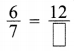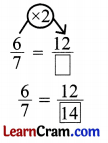# DAV Class 4 Maths Chapter 9 Worksheet 3 Solutions

The DAV Class 4 Maths Solutions and DAV Class 4 Maths Chapter 9 Worksheet 3 Solutions of Fractions offer comprehensive answers to textbook questions.

## DAV Class 4 Maths Ch 9 WS 3 Solutions

Question 1.
Find the missing numerator or denominator.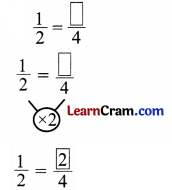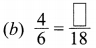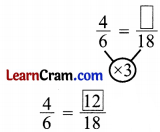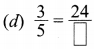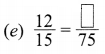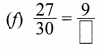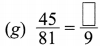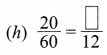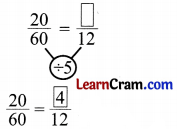Question 2.
Find the equivalent fractions of y with –
(a) Numerator 5
Numerator 5
$$\frac{1 \times 5}{3 \times 5}=\frac{5}{15}$$
= $$\frac{5}{15}$$

(b) Denominator 18
Denominator 18
$$\frac{1 \times 6}{3 \times 6}=\frac{6}{18}$$
= $$\frac{6}{18}$$

(c) Numerator 11
Numerator 11
$$\frac{1 \times 11}{3 \times 11}=\frac{11}{33}$$
= $$\frac{11}{33}$$

Question 3.
Change  into an equivalent fraction with denominator 54.
$$\frac{5 \times 6}{9 \times 6}=\frac{30}{54}$$
= $$\frac{30}{54}$$

Question 4.
Change into an equivalent fraction with numerator 5.
$$\frac{20 \div 4}{28 \div 4}=\frac{5}{7}$$
= $$\frac{5}{7}$$

### DAV Class 4 Maths Chapter 9 Worksheet 3 Notes

Missing Numerator Or Denominator Of Equivalent Fractions
Example 1:
Missing numerator of